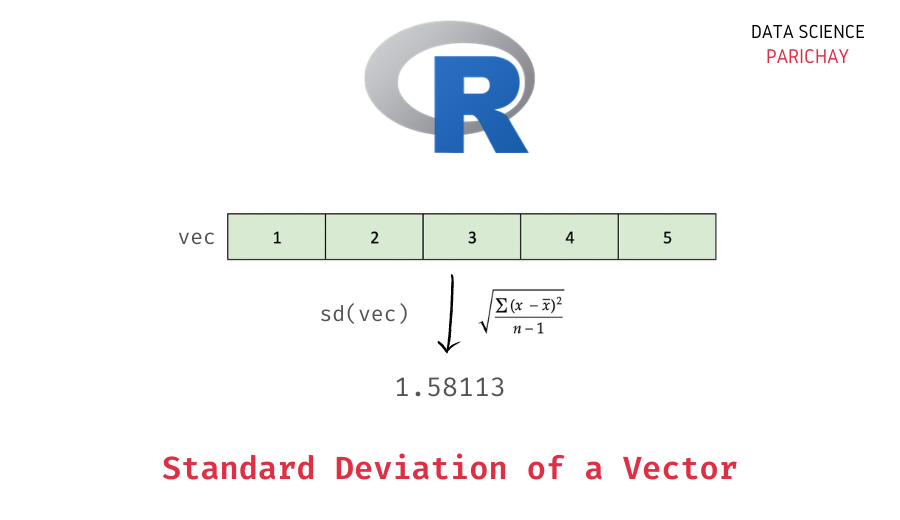# R – Get Standard Deviation of a Vector

R comes with a number of built-in functions to compute common descriptive statistics like the mean, median, variance, standard deviation, etc. In this tutorial, we will look at how to get the standard deviation of values in an R vector with the help of some examples.

## How to get the standard deviation of values in a vector in R?

You can use the R `sd()` function to get the standard deviation of values in a vector. Pass the vector as an argument to the function. The following is the syntax –

```# std deviation of values in a vector
sd(x, na.rm=FALSE)```

The following are the arguments that you can give to the `sd()` function in R.

• x – The vector for which you want to compute the standard deviation.
• na.rm – (Optional argument) Indicates whether to remove missing values before computing the standard deviation. It is `FALSE` by default.

The function returns the sample standard deviation of values in the passed vector.

## Examples

Let’s look at some examples of using the above method to get the standard deviation of a vector.

### Standard Deviation of values in a numeric vector

Let’s create a vector of numbers (and without any `NA` values) and apply the `sd()` function.

```# create a vector
vec <- c(1, 2, 3, 4, 5)
# std deviation of values in the vector
sd(vec)```

Output:

`1.58113883008419`

We get the standard deviation of the values in the above vector as 1.5811.

📚 Data Science Programs By Skill Level

Introductory

Intermediate ⭐⭐⭐

🔎 Find Data Science Programs 👨‍💻 111,889 already enrolled

Disclaimer: Data Science Parichay is reader supported. When you purchase a course through a link on this site, we may earn a small commission at no additional cost to you. Earned commissions help support this website and its team of writers.

### Standard Deviation of values in a vector with `NA` values

What would happen if there are some `NA` present values in the vector?

Let’s find out.

First, we will create a vector with some `NA` values and then apply the `sd()` function without any additional arguments.

```# create a vector with NA values
vec <- c(1, 2, NA, 3, 4, 5, NA)
# std deviation of values in the vector
sd(vec)```

Output:

`<NA>`

You can see that we get `NA` as the output. This is because performing an arithmetic operation with `NA` results in an `NA` in R.

You can pass `TRUE` to the `na.rm` parameter of the `sd()` function to exclude missing values when computing the standard deviation of a vector.

```# create a vector with NA values
vec <- c(1, 2, NA, 3, 4, 5, NA)
# std deviation of values in the vector
sd(vec, na.rm = TRUE)```

Output:

`1.58113883008419`

Now we get the standard deviation of the values in the above vector as 1.5811.

### Variance of values in a Vector

Standard deviation is defined as the square root of the variance. Thus, you can square the result from the `sd()` function in R using the `^` operator to get the variance. Let’s look at an example.

```# create a vector
vec <- c(1, 2, 3, 4, 5)
# variance of values in the vector
sd(var)^2```

Output:

`2.5`

Here, we get the variance of the values in the above vector as 2.5, which is the square of the standard deviation, 1.5811. Alternatively, you can also use the `var()` function in R to compute the variance directory without using the `sd()` function.

You might also be interested in –

•